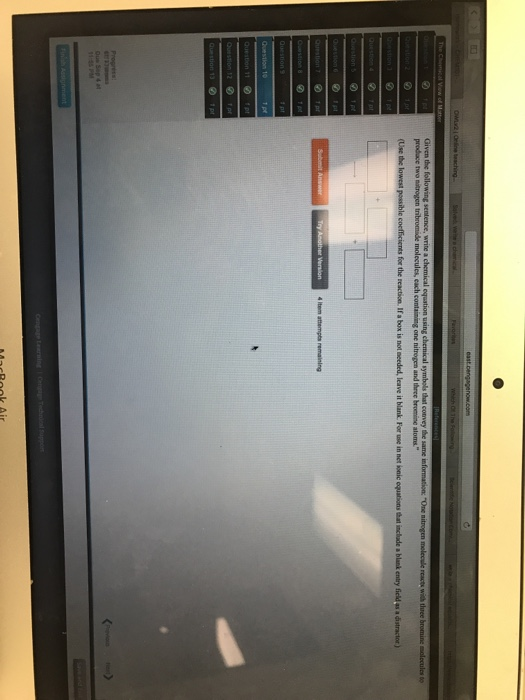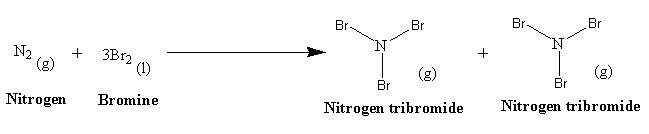# Question & Answer: Giving the following sentence, write a chemical equation using chemical symbols that convey…..Giving the following sentence, write a chemical equation using chemical symbols that convey the same information: “One nitrogen molecule reacts with three bromine molecules to produce two nitrogen tribromide molecules, each containing one nitrogen and three bromine atoms.” (Use the lowest possible coefficient for the reaction. If a box is not needed, leave it blanks. For use in net ionic equation that include a blank entry field as a distractor.)